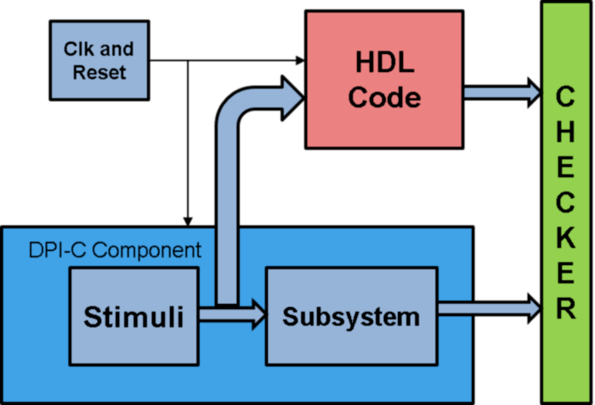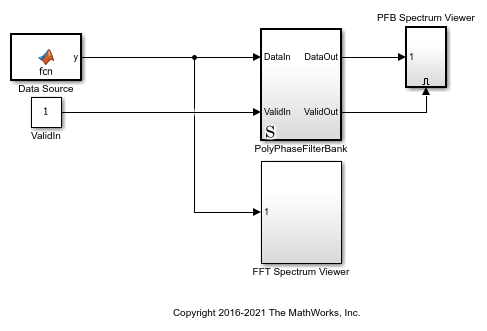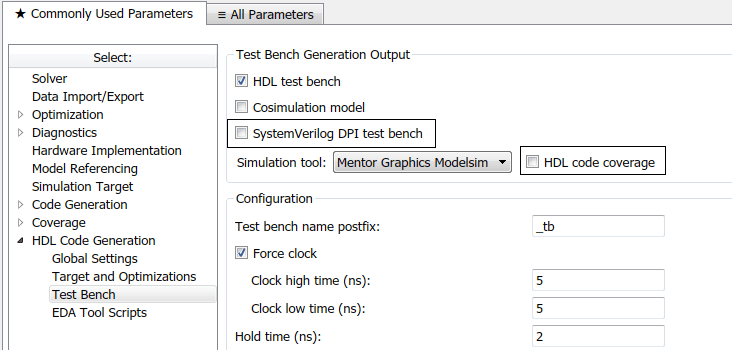# Verify HDL Design Using SystemVerilog DPI Test Bench

This example shows how to use SystemVerilog DPI test bench for verification of HDL code where a large data set is required.

In certain applications, simulation of a large number of samples is required to verify the HDL code generated by HDL Coder™ for your algorithm. For instance, these applications require a large number of samples for algorithm verification:

a) Calculation of radar astronomy frequency channels using a polyphase filter bank.

b) Obtaining the Bit Error Rate (BER) from a Viterbi decoder in a communications system.

c) Pixel-streaming video processing algorithms on high-resolution video.

Generating an HDL test bench to verify such a design is time consuming because the coder must simulate the model in Simulink® to capture the test bench data.

A faster generated test bench alternative is the HDL Verifier™ SystemVerilog DPI test bench. The SystemVerilog DPI test bench does not require a Simulink simulation, so for large data sets it generates a test bench in a shorter time than the HDL test bench.

HDL Verifier SystemVerilog DPI test bench integrates with Simulink Coder™ to export a Simulink system as generated C code inside a SystemVerilog component with a Direct Programming Interface (DPI). Within the DPI-C component the stimuli is generated and applied to the C subsystem and also applied to the generated HDL code for the Simulink system. The test bench compares the output of the HDL simulation with the output of the DPI-C component to verify the HDL design.### Polyphase Filter Bank

Polyphase filter bank is a widely used technique to reduce inaccuracy in FFT due to leakage and scalloping losses. A polyphase filter bank produces a flatter response as compared to a normal DFT by suppressing out-of-band signals significantly.

The model is a Polyphase Filter Bank which consists of a filter and an FFT that processes 16 samples at a time. For more information about the polyphase filter bank see High-Throughput Channelizer for FPGA.

```modelname = 'PolyphaseFilterBankHDLExample_4tap'; open_system(modelname); ```### Set Up the Model

The example model implements a 512-point FFT by using the DSP HDL Toolbox FFT block and a 4-tap filter for each band by using basic Simulink blocks. These MATLAB variables configure the blocks in the model. Use the dsp.Channelizer System object™ to generate the coefficients. The polyphase method of the channelizer object generates a 512-by-4 matrix. Each row represents the coefficients for one band. Cast the coefficients to fixed-point types that have the same word length as the input signal.

```simTime = 1000; FFTLength = 512; InVect = 4; h = dsp.Channelizer; h.NumTapsPerBand = 4; h.NumFrequencyBands = FFTLength; h.StopbandAttenuation = 60; coef4Tap = fi(polyphase(h),1,15,14,'RoundingMethod','Convergent'); % % The algorithm requires 512 filters (one filter for each band). For a % vector input of 4 samples, the model implements four parallel 4-tap % filters. Each filter applies 128 sets of coefficients. % ReuseFactor = FFTLength/InVect; % % These variables configure the model to pipeline the multipliers and % the coefficient bank to fit the logic into DSP blocks on the FPGA. Using % the DSP blocks enables synthesis to a higher clock rate. % Multiplication_PipeLine = 2; CoefBank_PipeLine = 1; % % The input data consists of two sine waves, 200 kHz and 250 kHz. The % input and output of the PolyPhaseFilterBank subsystem are 4-by-1 vectors. ```

### Generate HDL Code, HDL Test Bench, and SystemVerilog DPI Test Bench

Check the PolyphaseFilterBank subsystem for HDL code generation compatibility:

```checkhdl('PolyphaseFilterBankHDLExample_4tap/PolyPhaseFilterBank'); ```

Run the following command to generate HDL code:

```makehdl('PolyphaseFilterBankHDLExample_4tap/PolyPhaseFilterBank'); ```

Run the following command to generate the test bench:

```makehdltb('PolyphaseFilterBankHDLExample_4tap/PolyPhaseFilterBank'); ```

This command generates an HDL test bench by simulating the model in Simulink and then capturing the test bench data.

Run the following command to generate SystemVerilog DPI test bench:

```HDLSimulator = 'ModelSim'; % Supported Simulator Options = 'ModelSim', 'Incisive', 'VCS', 'Vivado' ```
```makehdltb('PolyphaseFilterBankHDLExample_4tap/PolyPhaseFilterBank', ... 'GenerateSVDPITestBench', HDLSimulator, ... 'GenerateHDLTestBench', 'Off'); ```

This command generates a SystemVerilog test bench without running a Simulink simulation. Instead of a simulation, the code exports the Simulink system as generated C code inside a SystemVerilog component. The test bench verifies the output data by comparing it with the output of the HDL design. The makehdltb function also generates simulator-specific scripts for compilation and simulation.

SystemVerilog DPI test bench can be used to verify HDL designs of both target languages - VHDL® and Verilog®.

Alternatively, you can set SystemVerilog DPI test bench options on the 'HDL Code Generation > Test Bench' pane in Configuration Parameters.### Generated SystemVerilog DPI Test Bench Artifacts

When you request a SystemVerilog DPI test bench, the coder generates the following artifacts:

a.) PolyPhaseFilterBank_dpi_tb.sv - This is the SystemVerilog test bench that verifies the HDL code.

b.) PolyPhaseFilterBank_dpi_tb.do - This is the macro file that Mentor Graphics ModelSim® uses to compile the HDL code and run the test bench simulation.

Based on the selected simulator, the coder generates a different file for compilation and test bench simulation. For instance, if you select 'Incisive', the coder generates 'PolyPhaseFilterBank_dpi_tb.sh' for compilation and simulation on Cadence® Incisive®.

### (Optional) Generate HDL Code Coverage Report and Database

To instrument the HDL Simulator to generate a HDL code coverage report and database, either:

a.) On the 'HDL Code Generation > Test Bench' pane, select the check box labeled 'HDL code coverage'.

b.) When you call 'makehdltb', set 'HDLCodeCoverage' to 'on'. For example:

```makehdltb('PolyphaseFilterBankHDLExample_4tap/PolyPhaseFilterBank', ... 'GenerateSVDPITestBench', HDLSimulator, ... 'GenerateHDLTestBench', 'Off', ... 'HDLCodeCoverage', 'On'); ```

The HDL code coverage artifacts are generated in the source directory after the test bench is simulated.

### Generation Time Comparison of HDL Test Bench and SystemVerilog DPI Test Bench

The example model runs for 1000 time steps, specified by the simTime variable. The sampling frequency is 2e+6 Hz, which means that the simulation to generate the HDL testbench collects 8e+9 samples.

For certain applications, it takes more samples to obtain the right frequency from the polyphase filter. An increase in simulation time also increases the time required to generate an HDL test bench.

A solution for such applications is to use the SystemVerilog DPI test bench. The generation time for the test bench remains the same no matter how many samples your test scenario requires.

You can increase the Simulation Time by changing the 'simTime' variable. For instance to generate an HDL test bench for 2e+12 samples, set:

```simTime = 1000000; ```

The table shows a comparison of time taken (in seconds) for generation of HDL test bench and SystemVerilog DPI test bench for increasing numbers of samples (from 2e+9 to 2e+15) :

```columns = {'NumberOfSamples';'GenerationTimeHDLTestBench';'GenerationTimeSystemVerilogDPITestbench'}; numSamples = [2e9;2e10;2e11;2e12;2e13;2e14;2e15]; HDLTBtime= [10;12;59;504;4994;52200;505506]; DPICTBtime=[47;47;47;47;47;47;47]; CompareTestBenchTimes = table(numSamples,HDLTBtime,DPICTBtime,'VariableNames',columns); disp(CompareTestBenchTimes); ```
``` NumberOfSamples GenerationTimeHDLTestBench GenerationTimeSystemVerilogDPITestbench _______________ __________________________ _______________________________________ 2e+09 10 47 2e+10 12 47 2e+11 59 47 2e+12 504 47 2e+13 4994 47 2e+14 52200 47 2e+15 5.0551e+05 47 ```

A log plot of generation time for both these test bench types with respect to the Number of samples, shows that while HDL test bench requires more generation time with an increase in the number of samples, generation time for the SystemVerilog DPI test bench remains constant irrespective of the number of samples.

```loglog(numSamples,HDLTBtime,'b-o', numSamples,DPICTBtime, 'r-o' ); xlim([2e09 2e15]); legend('HDL Test Bench','SystemVerilog DPI Test Bench','Location','northwest'); xlabel('Number Of Samples'); ylabel('Generation time (in seconds)'); ```### Conclusion

While HDL test bench is very efficient for a small number of samples, if your test scenario requires a large number of samples, HDL Verifier SystemVerilog DPI test bench provides faster test bench generation.